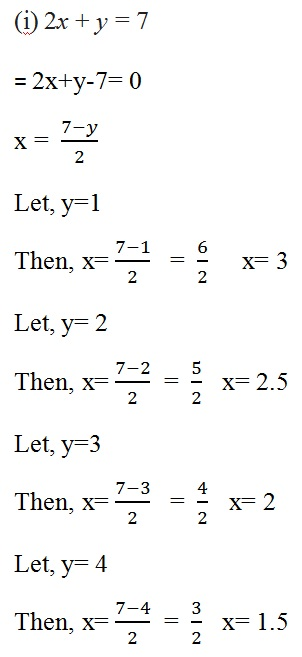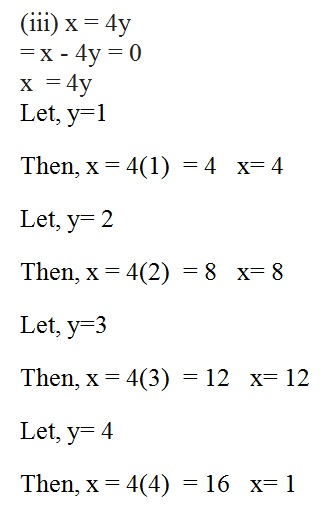Study Materials

# NCERT Solutions for Class 9th Mathematics

Page 2 of 4

## Chapter 4. Linear Equation In Two Variables

### Exercise 4.2

Chapter 4. Linear Equation In Two Variables

Exercise 4.2

1. Which one of the following options is true, and why?

y = 3x + 5 has

(i) a unique solution,  (ii) only two solutions,

Solution

(iii) infinitely many solution, because we can put many value of x and can get many solution of y.

2. Write four solutions for each of the following equations:

(i) 2x + y = 7

(ii) πx + y = 9

(iii) x = 4y

Solution3. Check which of the following are solutions of the equation – 2= 4 and which are not:

(i) (0, 2)

(ii) (2, 0)

(iii) (4, 0)Solution :

x = 4 + 2y

(i) (0,2)

Putting value of x and y

=  0 = 4 + 2(2)

=  0 = 4 + 4    =  0 = 8, hence it is not a solution of eq

(ii) (2,0)

Putting value of x and y

=  2 = 4 + 2(0)   =  2 = 4, hence it is also not a solution of eq

(iii) (4,0)

Putting value of x and y

=   4 = 4 + 2(0)    =  4 = 4, hence it is a solution of eq(v) (1,1)

Putting value of x and y

=   1 = 4 + 2(1)   = 1= 6, hence, it is not a solution of eq

4. Find the value of k, if = 2, = 1 is a solution of the equation 2+ 3k.

Solution :

2x + 3y = k

Putting the value of x and y

2(2) + 3(1) = k   = 4+3 = k    = k = 7

Page 2 of 4

Chapter Contents: